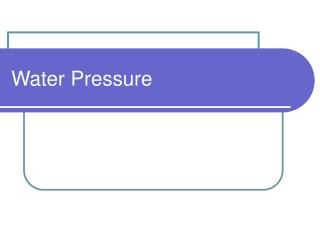DownloadDownload PresentationWater Pressure

# Water Pressure

Télécharger la présentation## Water Pressure

- - - - - - - - - - - - - - - - - - - - - - - - - - - E N D - - - - - - - - - - - - - - - - - - - - - - - - - - -
##### Presentation Transcript

1. Water Pressure

2. Water Pressure • Measured in pounds per square inch (psi) • Pressure expressed in units of feet of water where 1 psi = 2.31 feet of water • Desirable • Within most homes- approx. 40 psi

3. Friction Loss (head loss) • Friction Loss (head loss) • After loss, still need to be able to provide at least 40 psi (92.4 feet) Actual Pressure = Static Pressure – head loss (all expressed in psi) Static Pressure = (elevation of H20 level – elevation of water at outlet point) 2.31 ft/psi

4. Static Pressure Example: Determine the static pressure of a water tank sitting on your property that is 100 feet in the air and the base is at an elevation 2500 ft. Static Pressure = (elevation of H20 level – elevation of water at outlet point) 2.31 ft/psi

5. Static Pressure Example: Determine the static pressure of a water tank sitting on your property that is 100 feet in the air and the base is at an elevation 2500 ft. Static Pressure = (elevation of H20 level – elevation of water at outlet point) 2.31 ft/psi Static Pressure =2600 ft – 2500 ft = 43.3 psi 2.31 ft/psi

6. Greenville Example: Determine the static pressure (psi) of water for a storage tank in Greenville at an elevation of 2409 feet above the water-discharge elevation point of 2231 feet.

7. Greenville Example: Determine the static pressure (psi) of water for a storage tank in Greenville at an elevation of 2409 feet above the water-discharge elevation point of 2231 feet. Static Pressure = (elevation of H20 level – elevation of water at outlet point) 2.31 ft/psi = (2409 – 2231) / 2.31 = 77.06 psi

8. Greenville Example: Static Pressure: 77.06 psi **However, friction, or head losses, reduce the static pressure. **Hazen-Williams - calculate head loss due to friction in straight pipe

9. Hazen-Williams formula Hf = (10.44)(L)(Q1.85) (C1.85)(d4.8655) Hf = head loss due to friction, feet L = length of pipe, feet Q = flowrate of water through the pipe, gpm C = Hazen-Williams coefficient (on table) d = diameter of the pipe, inches

10. Hazen-Williams formula “Q” - Design Basis for Water Consumption Document “C” – Hazen-Williams coefficient; non-dimensional number that relates to the roughness of the pipe (See Specific Roughness and H-W Constants for Various Materials)

11. Pipe Systems • Most made of ductile iron (d.i.) or cast iron • 8 inches in diameter or greater • New, clean ductile iron pipe has a C = 130 • Over time, chemical reactions affect the roughness of the pipe. • C = 100 (existing pipe networks)

12. Greenville Example (cont.) Determine the head loss for a water distribution system that is a straight line from the Greenvillle water tower to a structure 4 miles away. The piping system is made with newly installed 8-inch ductile pipe. The flow rate has been determined to be 100 gallons per minute (gpm). Hf = (10.44)(L)(Q1.85) = (C1.85)(d4.8655)

13. Greenville Example (cont.) Determine the head loss for a water distribution system that is a straight line from the Greenvillle water tower to a structure 4 miles away. The piping system is made with newly installed 8-inch ductile pipe. The flow rate has been determined to be 100 gallons per minute (gpm). Hf = (10.44)(L)(Q1.85) = (10.44)(4●5238)(1001.85) = (C1.85)(d4.8655) (1001.85)(84.8655)

14. Greenville Example (cont.) Determine the head loss for a water distribution system that is a straight line from the Greenvillle water tower to a structure 4 miles away. The piping system is made with newly installed 8-inch ductile pipe. The flow rate has been determined to be 100 gallons per minute (gpm). Hf = (10.44)(4●5238)(1001.85) = 8.9 ft. (1001.85)(84.8655) = 8.9 ft of head loss because of the resistance to flow

15. Greenville Example (cont.) Now determine the actual pressure at the entrance to the structure. Actual Pressure = Static Pressure – head loss (all expressed in psi)

16. Greenville Example (cont.) Now determine the actual pressure at the entrance to the structure. Actual Pressure = Static Pressure – head loss (all expressed in psi) = 77.06 psi – 8.9 ft (1psi/2.31 ft) = 77.06 psi- 3.85 psi = 73.21 psi